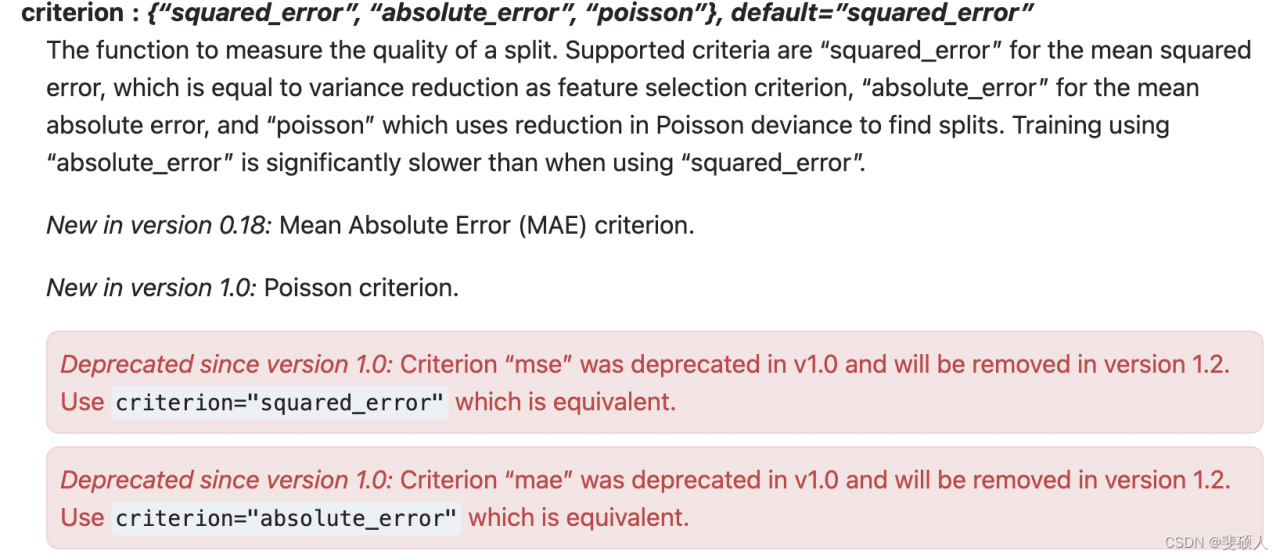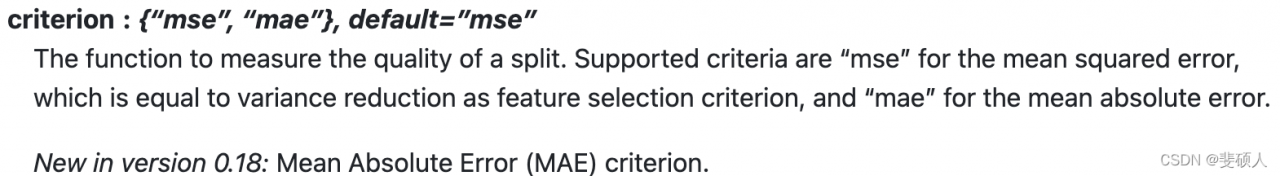# [Solved] fit function error: KeyError: ‘squared_error‘

critical code

``````param_grid_simple = {"criterion": ["squared_error","poisson"]
, 'n_estimators': [*range(20,100,5)]
, 'max_depth': [*range(10,25,2)]
, "max_features": ["log2","sqrt",16,32,64,"auto"]
, "min_impurity_decrease": [*np.arange(0,5,10)]
}
search = GridSearchCV(estimator=reg
,param_grid=param_grid_simple
,scoring = "neg_mean_squared_error"
,verbose = True
,cv = cv
,n_jobs=-1)
search.fit(X,y)
``````

Error reporting information

``````~/anaconda3/lib/python3.8/site-packages/sklearn/ensemble/_forest.py in _parallel_build_trees(tree, forest, X, y, sample_weight, tree_idx, n_trees, verbose, class_weight, n_samples_bootstrap)
166                                                         indices=indices)
167
--> 168         tree.fit(X, y, sample_weight=curr_sample_weight, check_input=False)
169     else:
170         tree.fit(X, y, sample_weight=sample_weight, check_input=False)

~/anaconda3/lib/python3.8/site-packages/sklearn/tree/_classes.py in fit(self, X, y, sample_weight, check_input, X_idx_sorted)
1240         """
1241
-> 1242         super().fit(
1243             X, y,
1244             sample_weight=sample_weight,

~/anaconda3/lib/python3.8/site-packages/sklearn/tree/_classes.py in fit(self, X, y, sample_weight, check_input, X_idx_sorted)
334                                                          self.n_classes_)
335             else:
--> 336                 criterion = CRITERIA_REG[self.criterion](self.n_outputs_,
337                                                          n_samples)
338

KeyError: 'squared_error'
``````

analysis

KeyError error will be caused when accessing a key that is not in dict, then the parameter of ` criterion` is ` squared_error` may not exist. Since the parameter value is known, it is speculated that there may be a problem with your own sklearn version. Check your version of sklearn is 0.23, while the official version has already been above 1.0.

Solution:

See the official document of sklearn

scikit-learn 1.1. dev0scikit-learn 0.23.2You can see that different versions of the criterion parameter have different values, which can be considered

1. Change the value to the value of the corresponding version, such as’ MSE ‘.

2. Change the version of sklearn directly.

Because the official document says, “MSE” is in V1.0 has been deprecated and will be removed in version 1.2. “Squared_error” is equivalent. Therefore, the method of upgrading sklearn is adopted.

``````pip install scikit-learn==1.0.1
``````